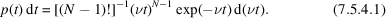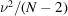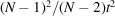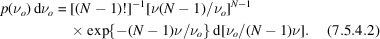International
Tables for
Crystallography
Volume C
Mathematical, physical and chemical tables
Edited by E. Prince

International Tables for Crystallography (2006). Vol. C, ch. 7.5, p. 667

## Section 7.5.4. Fixed-count timing

A. J. C. Wilsona

aSt John's College, Cambridge CB2 1TP, England

### 7.5.4. Fixed-count timing

| top | pdf |

The probability of a time t being required to accumulate N counts when the true counting rate is ν is given by a Γ distribution (Abramowitz & Stegun, 1964, p. 255):The ratio N/t is a slightly biased estimate of the counting rate ν; the unbiased estimate is (N − 1)/t. The variance of this estimate is, or, nearly enough for most purposes,. The differences introduced by the corrections −1 and −2 are generally negligible, but would not be so for counts as low as those proposed by Killean (1967). If such corrections are important, it should be noticed that there is an ambiguity concerning N, depending on how the timing is triggered. It may be triggered by a count that is counted, or by a count that is not counted, or may simply be begun, independently of the incidence of a count. Equation (7.5.4.1)assumes the first of these.

Equation (7.5.4.1)may be inverted to give the probability distribution of the observed counting rate νo instead of the probability distribution of the time t:There does not seem to be any special name for the distribution (7.5.4.2). Only its first (N − 1) moments exist, and the integral expressing the probability distribution of the difference of the reflection and the background rates is intractable (Wilson, 1980).

### References

Abramowitz, M. & Stegun, I. A. (1964). Handbook of mathematical functions. National Bureau of Standards Publication AMS 55.
Killean, R. C. G. (1967). A note on the a priori estimation of R factors for constant-count-per-reflection diffractometer experiments. Acta Cryst. 23, 1109–1110.
Wilson, A. J. C. (1980). Relationship between observed' and true' intensity: effect of various counting modes. Acta Cryst. A36, 929–936.